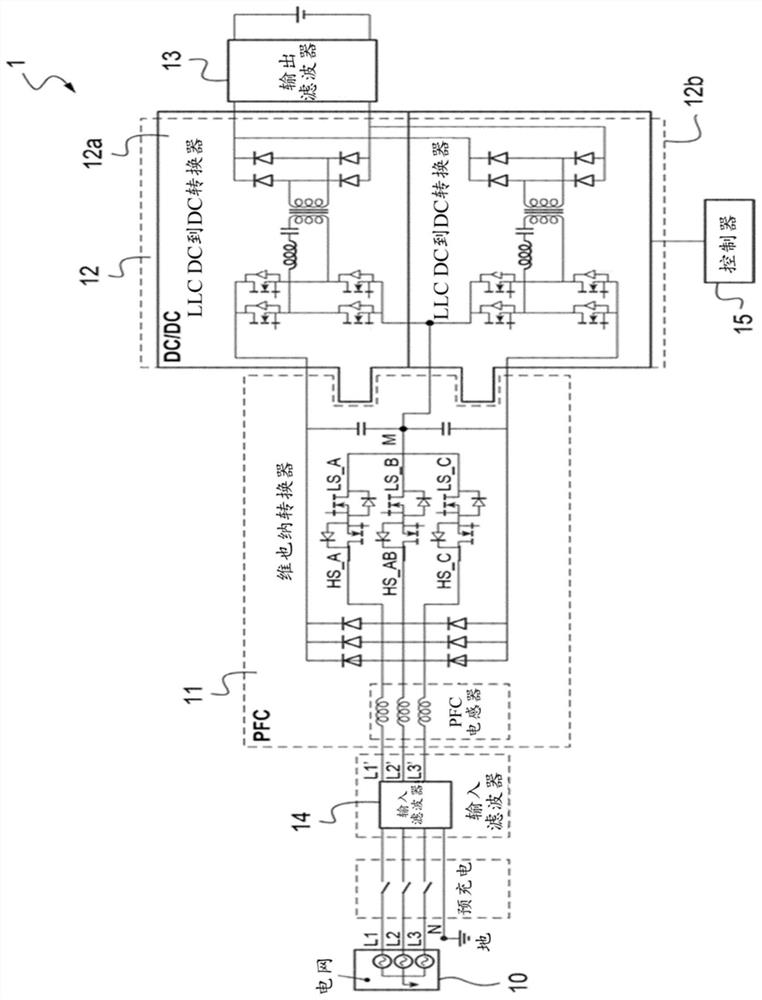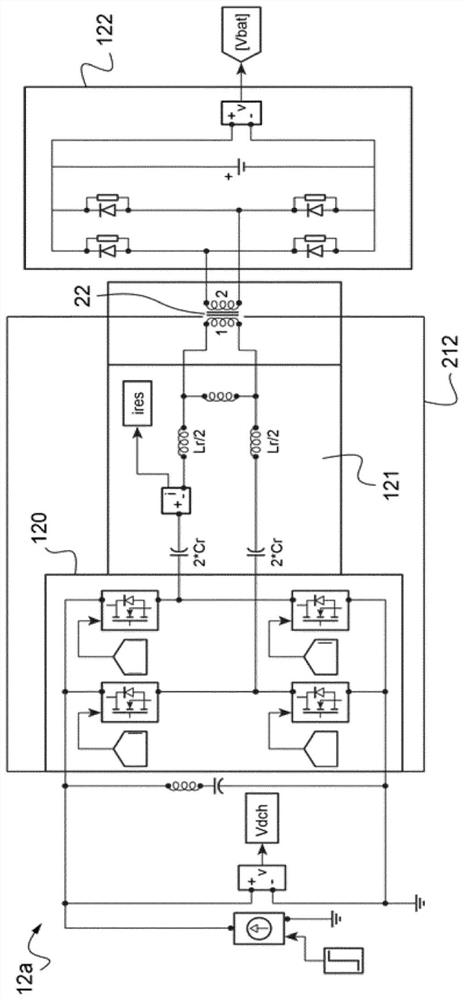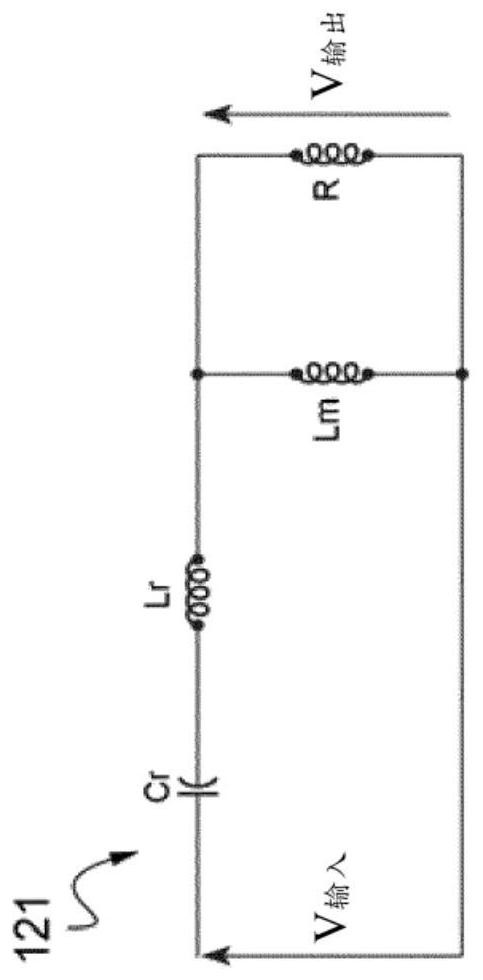# Method for controlling the input voltage frequency of a dc-dc convertor

## A technology for controlling frequency and current converters, used in converter types, conversion of DC power input to DC power output, control/regulation systems, etc., and can solve problems such as inability to provide solutions

Pending Publication Date: 2021-03-05
RENAULT SAS
0 Cites 0 Cited by

## AI-Extracted Technical Summary

### Problems solved by technology

 However, controlling the DC voltage at the input of an LLC DC to DC convert...
View more

## Abstract

The invention concerns a method (30) for controlling the input voltage frequency of a DC-DC converter (12) comprising a step (65) of calculating a control frequency value (F) of said DC-DC converter (12), wherein: - if the measured voltage (VDCM) is greater than said upper voltage limit (VDCR+eps), the control frequency (F) corresponds to said minimum control frequency (FRMIN); - if the measured voltage (VDCM) is less than said lower voltage limit (VDCR-eps), the control frequency corresponds to said maximum control frequency (FRMAX); and - if the measured voltage (VDCM) is between said uppervoltage limit (VDCR+eps) and said lower voltage limit (VDCR-eps), the control frequency (F) corresponds to an average frequency (FMOY) calculated as a function of the difference between the setpoint voltage value (VDCR) and the measured voltage (VDCM), upper error values (eps) and lower error values (-eps) and maximum (FRMAX) and minimum (FRMIN) control frequency values.

Application Domain

Charging stationsDc-dc conversion +7

Technology Topic

Dc converterConverters +3

## Image

•••## Examples

• Experimental program(1)

### Example Embodiment

Figure 1 to 6 It is related to the same embodiment and will be annotated simultaneously.
referencefigure 1 The charger 1 of the battery 13 connected to the three-phase grid 10 includes a power factor correction stage 11 (also referred to as PFC level 11) and DC current to DC current converter DC to DC 12A and 12B, each converter has inverter. The device 212.
The three-phase grid 10 is mounted on the input filter 14 which transfers the filtered input current to the PFC level 11.
At the output of the PFC 11, two DC voltage bus connected to the terminal of the PFC stage 11 are coupled to the DC to the DC converters 12a, 12b, respectively, and the two converters are connected in parallel with the battery 13.
Each DC to DC 12A, 12B (figure 2 Only one example is shown) includes input MOSFET bridge 120, and the LLC circuit 121 (which simplifies circuit diagram, such asimage 3 The transformer 22 and the output diode bridge 122 are shown.
The charger 1 also includes means 15 for controlling the DC current to the DC current converter 12, which can perform a control method 60 according to the present invention.
The control method 60 according to the present invention is intended to control the frequency of the input voltage of the DC current to the DC current converter 12.
Refer to Figure 4,Figure 5 withFigure 6 The method for controlling the DC current to the DC current converter includes a plurality of preparation steps 61, 62, 63. These preparation steps 61 to 63 are independent of each other. Preparation steps 61 to 63 are intended to define the operational parameters of the method; they can be performed before the method (e.g., at the calibration phase), or dynamically executed when the method begins.
These preparation steps 61 to 63 can also be reproduced during operation of method 60 to dynamically modify the operational parameters of the method.
First, implement the 61 maximum control frequency value FRMax And minimum control frequency value frMIN Steps, for example, in this case, maximum frequency frMax 200kHz, and minimum frequency FRMIN It is 60kHz.
Then implement the definition 62 set point voltage value VDCR The step of the input voltage should be converged to the set point voltage value. inFigure 5 Exemplary embodiments, VDCR = 450V.
Then define 63 error zone 51 defined by two error values ​​(upper error values ​​EPS and lower error value-EPS), which makes it possible to define an upper voltage value V.DRC + EPS and lower limit voltage value VDCR -EPS.
In this exemplary embodiment, error voltage + EPS = 100 V and -EPS = -100V is defined.
Frame setting point voltage VDCR These upper limit voltage values ​​VDRC + EPS and lower limit voltage value VDCR -EPS thus defines the set point voltage VDCR The amplitude of the surrounding error.
In this embodiment, the upper error value EPS and the lower error value-EPS have the same absolute value to set the point voltage value V.DCR A symmetrical error zone is defined around. However, the present invention is not limited to these same absolute values, and may specify that the upper error value EPS and the lower error value-EPS have different absolute values.
Then, the method implements the 64 input voltage VDCM The steps of the measured value.
The step of calculating the control frequency value of the 65DC current to the DC current converter is performed.
In this calculation step 65, the measured input voltage VDCM Value Valve Valve with the upper limit VDCR + EPS and lower limit voltage value VDCR -EPS is compared.
If the measured voltage VDCM More than or equal to the upper voltage VDCR + EPS, is applied equal to the minimum control frequency FRMIN Control frequency F.
If the measured voltage VDCM Small than or equal to the lower limit voltage VDCR -EPS, it is applied equal to maximum control frequency FR.Max Control frequency F.
Finally, if the measured voltage VDCM Strictly intermodal voltage VDCR + EPS with the lower limit voltage VDCR -EPS, the control frequency F corresponds to the average frequency f based on the value of the measurement voltage and the value of the set point voltage, and the value calculated by the maximum control frequency and the minimum control frequency.Moy.
This average frequency fMoy Use the following equation to calculate:

among them:
- Value error corresponds to the set point voltage value VDCR And measured voltage VDCM The difference, ie, error = VDCR -VDCM.
In other words, a pair of error parameters - EPS, EPS makes it possible to set point VDCR The vicinity defines the error zone, in which the control device calculates the accurate convergence point value V.DCR Frequency fMoy.
Specifically, when error VDRC -VDRMWhen one of the thresholds-Eps, EPS is reached, the control frequency is calculated to accurately reach the set point and eliminate the static error.
As described below, when it is higher than or below the error zone, the minimum frequency FR is applied.MIN Or maximum frequency frMax To ensure effective convergence.
ReferFigure 4A , When the voltage V is measuredDCM Strictly mediate the upper voltage VDCR + EPS with the lower limit voltage VDCR When the -EPS is between, the proportional integral controller 42 is activated, and is often referred to as a PI controller. This makes it possible to refine the calculation of the frequency f to be applied, and increase the convergence of the measured voltage to several volts.
Therefore, the output of the first control stage 41 is reached as a so-called feedforward open loop control command, and 43 is added to the result obtained by the PI controller 42.

## PUM## Description & Claims & Application Information

We can also present the details of the Description, Claims and Application information to help users get a comprehensive understanding of the technical details of the patent, such as background art, summary of invention, brief description of drawings, description of embodiments, and other original content. On the other hand, users can also determine the specific scope of protection of the technology through the list of claims; as well as understand the changes in the life cycle of the technology with the presentation of the patent timeline. Login to view more.
Who we serve
• R&D Engineer
• R&D Manager
• IP Professional
Why Eureka